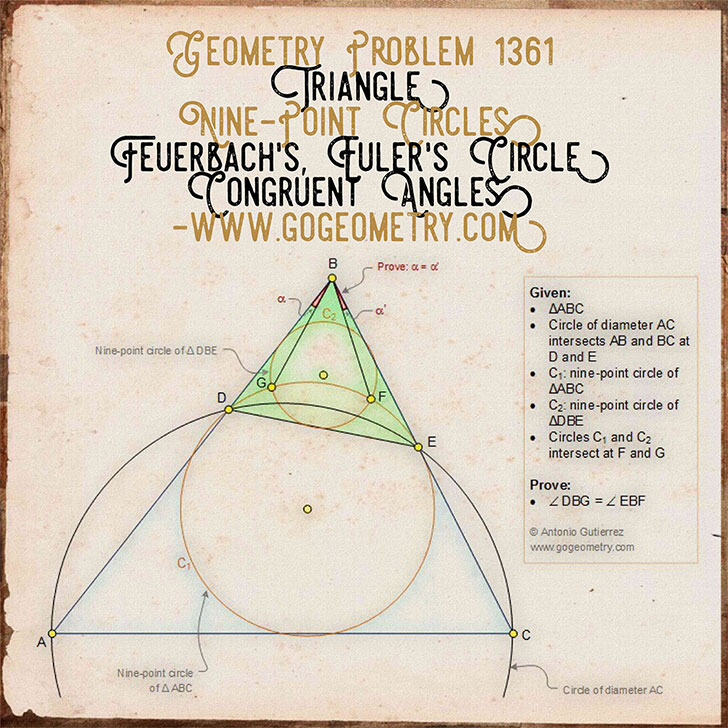# Geometry Problem 1361: Triangle, Two Nine-Point Circles, Feuerbach's Circle, Euler's Circle, Congruent Angles, Sketch, iPad Apps.

### Proposition

The figure below shows a triangle ABC. Circle of diameter AC intersects AB and BC at D and E, respectively. C1 is the nine-point circle of triangle ABC and C2 is the nine-point circle of triangle DBE. Circles C1 and C2 intersect at F and G. Prove that the angles DBG and EBF are congruent.### Sketch and Typography of problem 1361 using iPad Apps